Courses

# Past Year Questions: Traffic Engineering - 2 Civil Engineering (CE) Notes | EduRev

## Civil Engineering (CE) : Past Year Questions: Traffic Engineering - 2 Civil Engineering (CE) Notes | EduRev

The document Past Year Questions: Traffic Engineering - 2 Civil Engineering (CE) Notes | EduRev is a part of the Civil Engineering (CE) Course Topic wise GATE Past Year Papers for Civil Engineering.
All you need of Civil Engineering (CE) at this link: Civil Engineering (CE)

Question 1. The critical flow ratios for a three-phase signal are found to be 0.30, 0.25, and 0.25. The total time lost in the cycle is 10 s. Pedestrian crossings at the junction are not significant. The respective Green times (expressed in seconds and rounded off to the nearest integer) for the three phases are    [2016 : 2 Marks, Set-II]
(a) 34, 28 and 28
(b) 40, 25, and 25
(c) 40, 30 and 30
(d) 50, 25, and 25
(a)
Solution: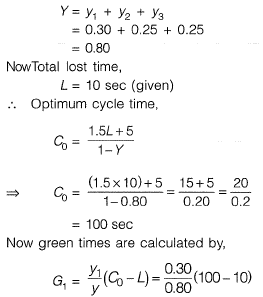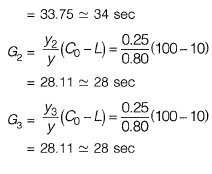Question 2.  If the total number of commercial vehicles per day ranges from 3000 to 6000, the minimum percentage of commercial traffic to be surveyed for axle load is     [2016 : 1 Mark, Set-II]
(a) 15
(b) 20
(c) 25
(d) 30
(a)

Question 3. While traveling along and against the traffic stream, a moving observer measured the relative flows as 50 vehicles/hr and 200 vehicles/hr, respectively. The average speeds of the moving observer while traveling along and against the stream are 20 km/hr and 30 km/hr, respectively. The density of the traffic stream (expressed in vehicles/km) is________.      [2016 : 2 Marks, Set-I]
Solution:

Method-I
Let us assume,
q = traffic flow
k = traffic density
V = velocity of test vehicle along the stream
V - velocity of test vehicle against the stream
=> q - KVw =50
=> q - k x 20 = 50 ...(i)
q + kVa = 200
=> q + k x 30 = 200 ...(ii)
Solving equation (i) and (ii), we get
k = 3 Veh/km
Method-ll
Consider a ‘L’ km long stretch,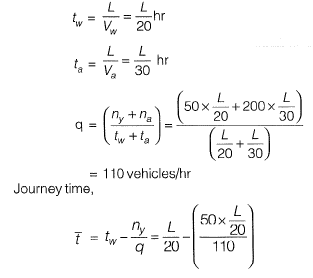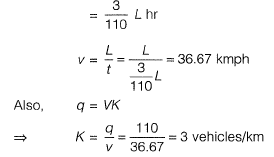Question 4. Inaone-lane one-way homogeneous traffic stream, the observed average headway is 3.0s. The flow (expression vehicles/hr) in this traffic stream is __________    [2016 : 1 Mark, Set-I]
Solution:
= 3.0 sec
Flow of traffic stream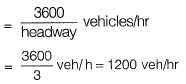Quesion 5. The relation between speed u(in km/h) and density k (number of vehicles/km) for a traffic stream on a road is u = 70 - 0.7k. The capacity on this road is ________ vph (vehicles/hour).     [2015 : 2 Marks, Set-II]
Solution:
Traffic volume,
q= uk
q= (70-0.7 k)k
= 70k-0.7 k2
Maximum possible volume is capacity of road
For maximum volume,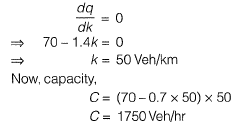Question 6. The following statements are made related to the lengths of turning lanes at signalised intersections
(i) 1.5 times the average number of vehicles (by vehicle type) that would store in turning lane per cycle during the peak hour.
(ii) 2 times the average number of vehicles (by vehicle type) that would store in turning lane per cycle during the peak hour.
(iii) Average number of vehicles (by vehicle type) that would store in the adjacent through lane per cycle during the peak hour.
(iv) Average number number of vehicles (by vehicle type) that would store in all lanes per cycle during the peak hour.
As per the IRC recommendations, the correct choice for design length of storage lanes is    [2015 :1 Mark, Set-Il]
(a) Maximum of (ii) and (iii)
(b) Maximum of (i) and (iii)
(c) Average of (i) and (iii)
(d) Only (iv)

Solution: Design length is the average of (i) and (iii) as per Indian Roads Congress (IRC) recommendations for storage lanes.

Question 7.  Which of the following statements CANNOT be used to describe free flow speed (uf) of a traffic stream?    [2015 : 1 Mark, Set-I]
(a) uf is the speed when flow is negligible
(b) uf is the speed when density is negligible
(c) uf is affected by geometry and surface conditions of the road
(d) uf is the speed at which flow is maximum and density is optimum

Solution: Free flow speed (Vf) is that speed at which flow and density is negligible.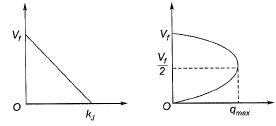Question 8.  A pre-timed four phase signal has critical lane flow rate for the first three phases as 200, 187 and 210 veh/hr with saturation flow rate of 1800 veh/hr/lane for all phases. The lost time is given as 4 seconds for each phase. If the cycle length is 60 seconds, the effective green time (in seconds) of the fourth phase i s ________ .     [2014 : 2 Marks, Set-II]
Solution:
Flow rates for the first three phase are given as
q1 = 200 veh/hr
q2 = 187 veh/hr
and q3 = 210 veh/hr
Saturation flow rate is 1800 veh/hr/lane
Lost time,
L = 4 x 4 = 16 sec
Length of the cycle,
C0 = 60 sec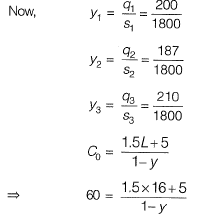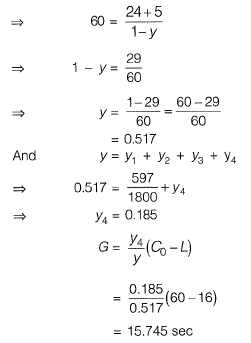Question 9. On a section of a highway the speed- density relationship is linear and is given by v = [80 - (2/3) k]; where v is in km/h and k is in veh/km. The capacity (in veh/h) of this section of the highway would be    [2014 : 2 Marks, Set-II]
(a) 1200
(b) 2400
(c) 4800
(d) 9600

Solution:
Method-I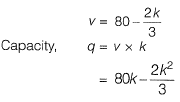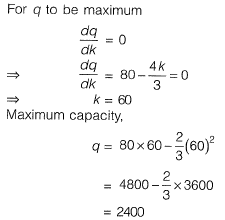Method-II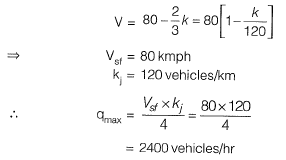Question 10. A student riding a bicycle on a 5 km one-way street takes 40 minutes to reach home. The student stopped for 15 minutes during this ride. 60 vehicles overtook the student (assume the number of vehicles overtaken by the student is zero) during the ride and 45 vehicles while the student stopped. The speed of vehicle stream on that road (in km/hr) is     [2014 : 2 Marks, Set-II]
(a) 7.5
(b) 12
(c) 40
(d) 60
Solution:

Method-I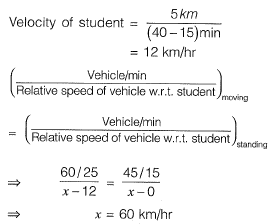Method-I
45 vehicles overtook the student when he stopped for 15 minutes.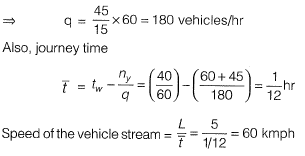Offer running on EduRev: Apply code STAYHOME200 to get INR 200 off on our premium plan EduRev Infinity!

67 docs

,

,

,

,

,

,

,

,

,

,

,

,

,

,

,

,

,

,

,

,

,

;